# CONTROL STRUCTURES Program instructions can be executed n

• Slides: 56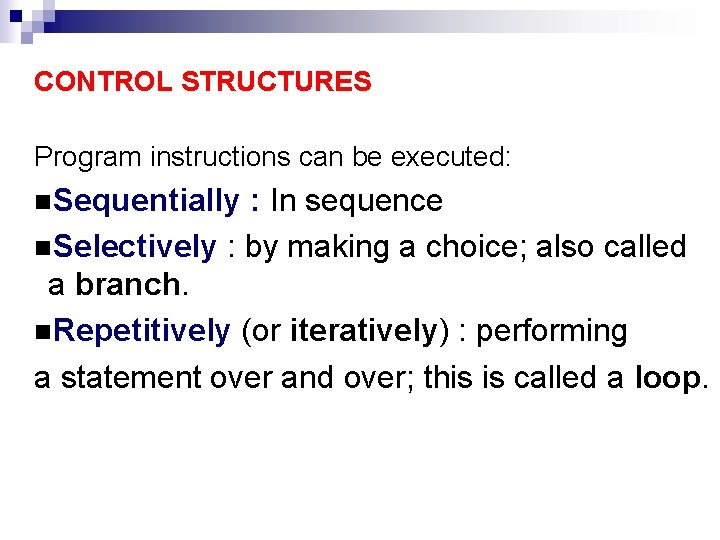CONTROL STRUCTURES Program instructions can be executed: n. Sequentially : In sequence n. Selectively : by making a choice; also called a branch. n. Repetitively (or iteratively) : performing a statement over and over; this is called a loop.expression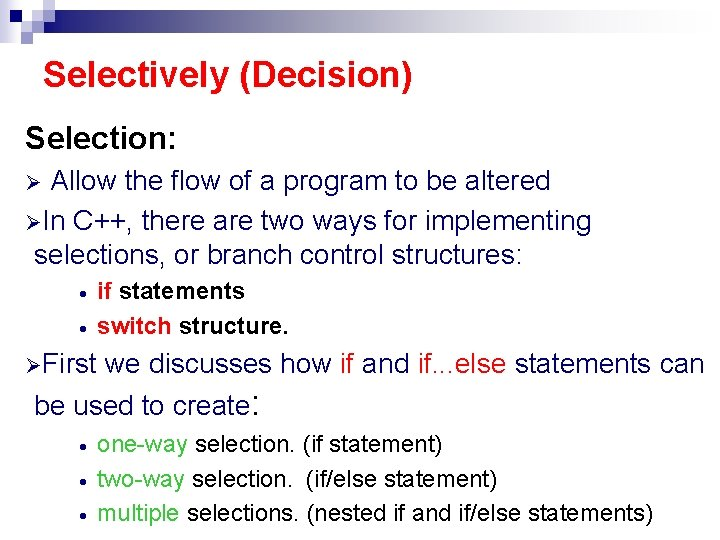Selectively (Decision) Selection: Allow the flow of a program to be altered ØIn C++, there are two ways for implementing selections, or branch control structures: Ø · · if statements switch structure. ØFirst we discusses how if and if. . . else statements can be used to create: · · · one-way selection. (if statement) two-way selection. (if/else statement) multiple selections. (nested if and if/else statements)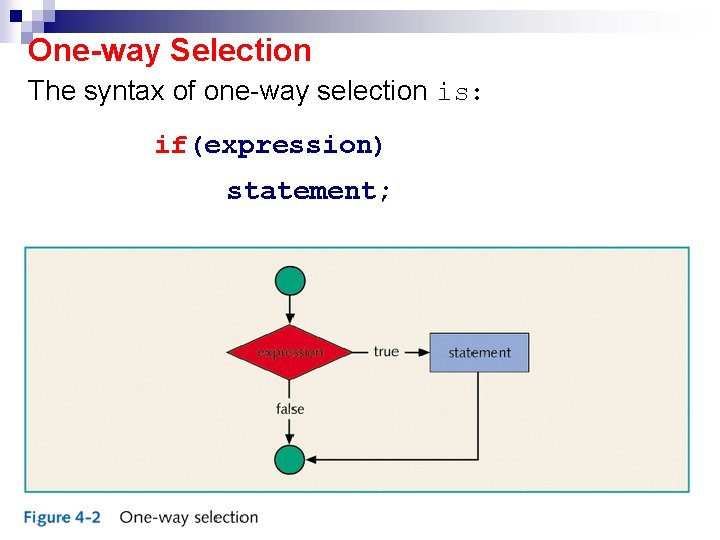One-way Selection The syntax of one-way selection is: if(expression) statement;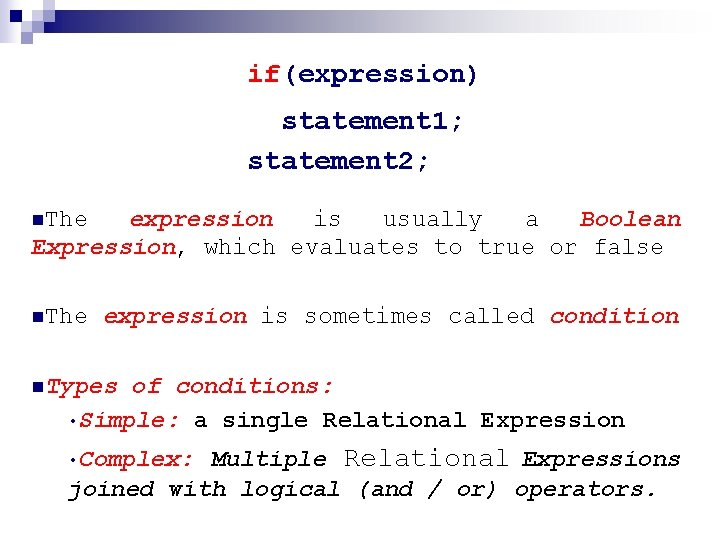if(expression) statement 1; statement 2; n. The expression is usually a Boolean Expression, which evaluates to true or false n. The expression is sometimes called condition n. Types of conditions: • Simple: a single Relational Expression Multiple Relational Expressions joined with logical (and / or) operators. • Complex:if(expression) statement 1; statement 2; n. If the value of the expression is true, statement 1 is executed then statement 2 nif the value is false, statement 1 is not executed and the computer goes on to the next statement (statement 2) in the program. nstatement n is any type of C++ statements. In C++, if is a reserved word.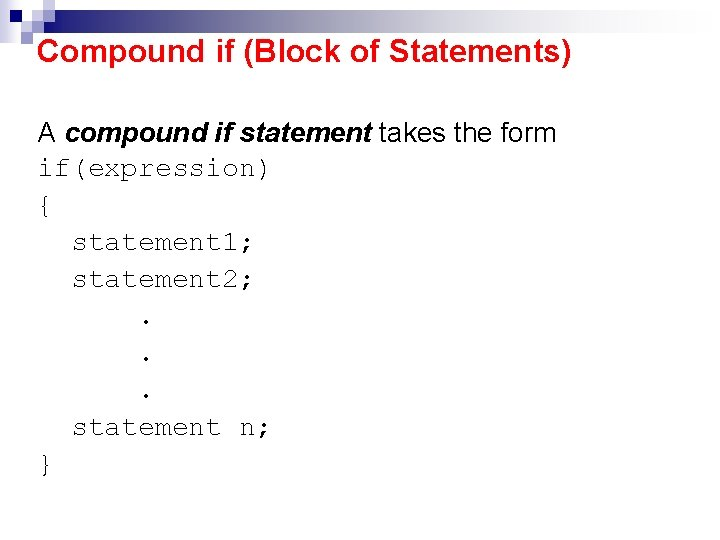Compound if (Block of Statements) A compound if statement takes the form if(expression) { statement 1; statement 2; . . . statement n; }Example # 1 if(score >= 90) grade = 'A'; If (score >= 90) is true, the assignment statement is executed; if it is false, the assignment statement is not executed. n For example, if the value of score is 95, the value assigned to the variable grade is A. n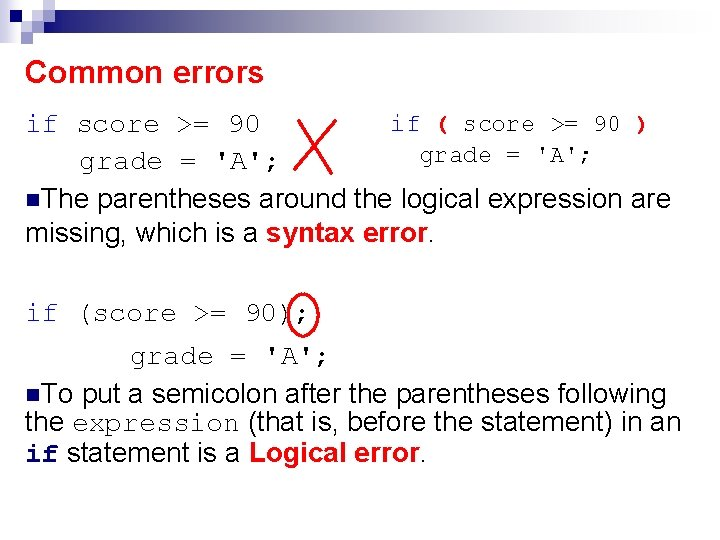Common errors if ( score >= 90 ) if score >= 90 grade = 'A'; n. The parentheses around the logical expression are missing, which is a syntax error. if (score >= 90); grade = 'A'; n. To put a semicolon after the parentheses following the expression (that is, before the statement) in an if statement is a Logical error.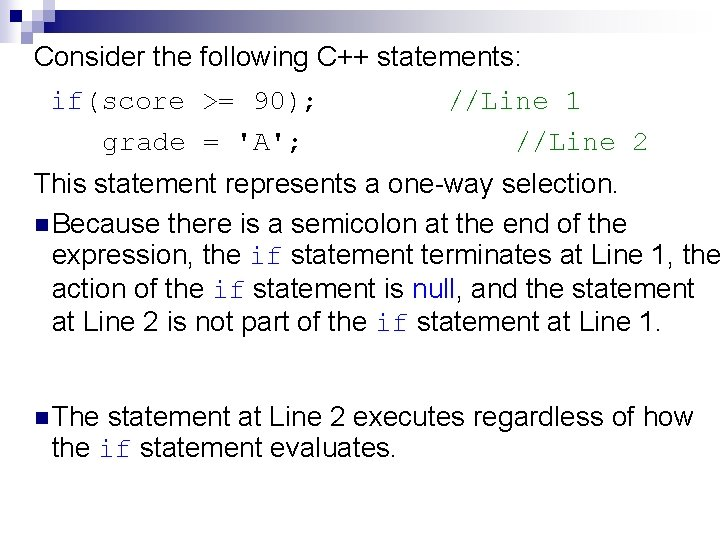Consider the following C++ statements: if(score >= 90); grade = 'A'; //Line 1 //Line 2 This statement represents a one-way selection. n Because there is a semicolon at the end of the expression, the if statement terminates at Line 1, the action of the if statement is null, and the statement at Line 2 is not part of the if statement at Line 1. n The statement at Line 2 executes regardless of how the if statement evaluates.Example # 2 Draw a flowchart and write a C++ program that finds the absolute value of an integer number. Start Flow chart: Read Number < 0 True number= -number False Write number EndExample#2 Program Code: void main () { int number; cout<<"Please enter an integer: "; cin>>number; if(number < 0) number = -number; cout<<endl<<"The absolute value is " <<number<<endl; getch(); } Please enter an integer -6734 The absolute value is 6734Two-way Selection The syntax of two-way selection is: if(expression) statement 1; else statement 2;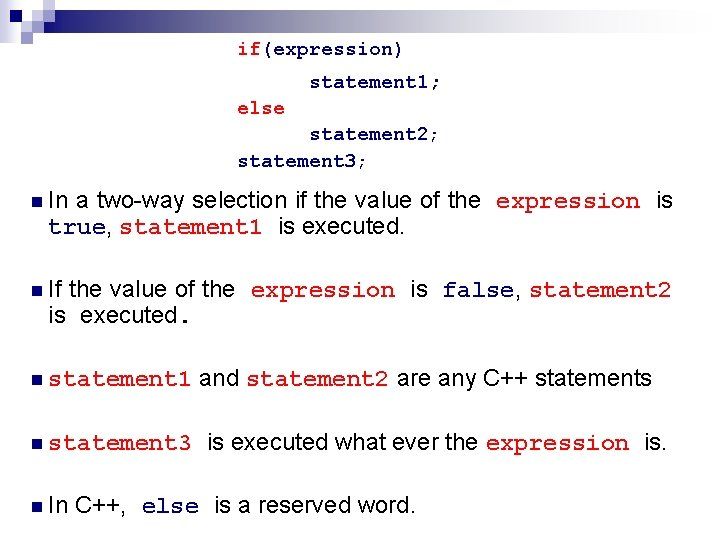if(expression) statement 1; else statement 2; statement 3; n In a two-way selection if the value of the expression is true, statement 1 is executed. n If the value of the expression is false, statement 2 is executed. n statement 1 n statement 3 n In and statement 2 are any C++ statements is executed what ever the expression is. C++, else is a reserved word.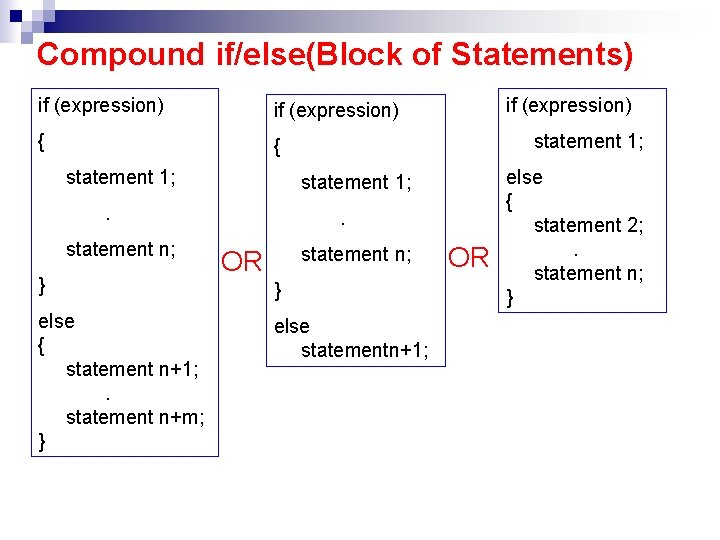Compound if/else(Block of Statements) if (expression) { { statement 1; } else { statement n+1; . statement n+m; } statement 1; . statement n; if (expression) . statement n; OR } else statementn+1; OR else { statement 2; . statement n; }Example#3 if(hours > 40. 0) salary = 40. 0 * rate + 100; else salary = hours * rate; //Line 1 2 3 4 n If the value of the variable hours is greater than 40. 0, then the salary include overtime payment. n Suppose that hours is 50. The expression in the if statement at Line 1 evaluates to true, so the statement at Line 2 executes. n If hours is 30, or any number less than or equal to 40, the expression in the if statement at Line 1 evaluates to false. The program skips the statement at Line 2 and executes the statement at Line 4.if(expression); ; Misplaced else statement 1; else statement 2; n To put a semicolon after the expression before statement 1, in a two-way selection, is a syntax error. In this case the if statement ends with the semicolon, statement 1 is no longer part of the if statement and the else part of the statement is all by itself. n Remember there is no else statement in C++. It cannot be separated from the if statement.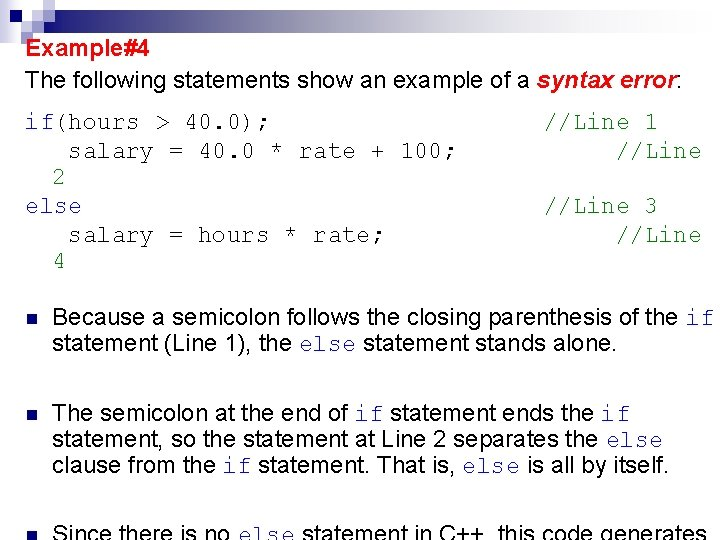Example#4 The following statements show an example of a syntax error: if(hours > 40. 0); salary = 40. 0 * rate + 100; 2 else salary = hours * rate; 4 //Line 1 //Line 3 //Line n Because a semicolon follows the closing parenthesis of the if statement (Line 1), the else statement stands alone. n The semicolon at the end of if statement ends the if statement, so the statement at Line 2 separates the else clause from the if statement. That is, else is all by itself.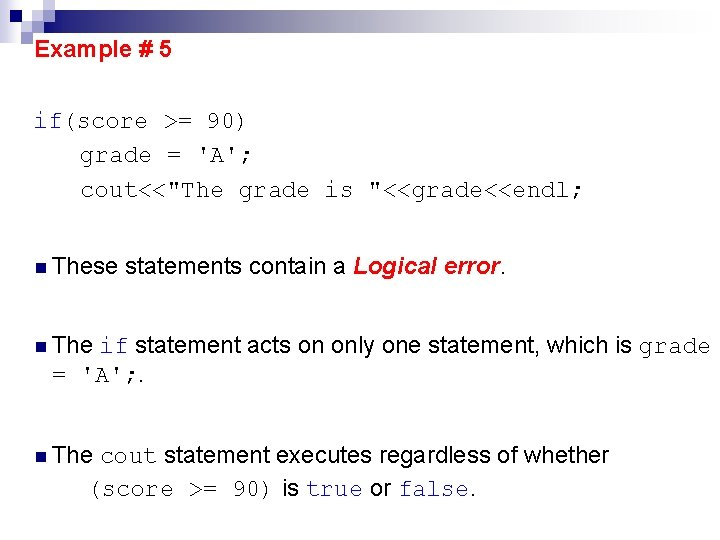Example # 5 if(score >= 90) grade = 'A'; cout<<"The grade is "<<grade<<endl; n These statements contain a Logical error. n The if statement acts on only one statement, which is grade = 'A'; . n The cout statement executes regardless of whether (score >= 90) is true or false.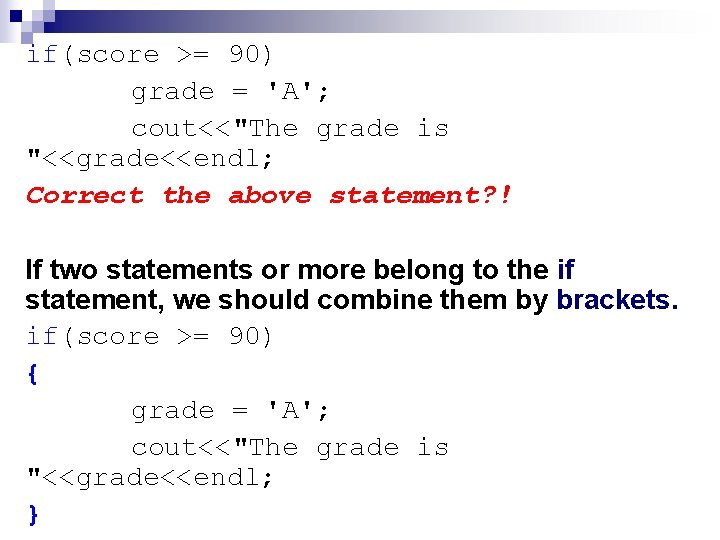if(score >= 90) grade = 'A'; cout<<"The grade is "<<grade<<endl; Correct the above statement? ! If two statements or more belong to the if statement, we should combine them by brackets. if(score >= 90) { grade = 'A'; cout<<"The grade is "<<grade<<endl; }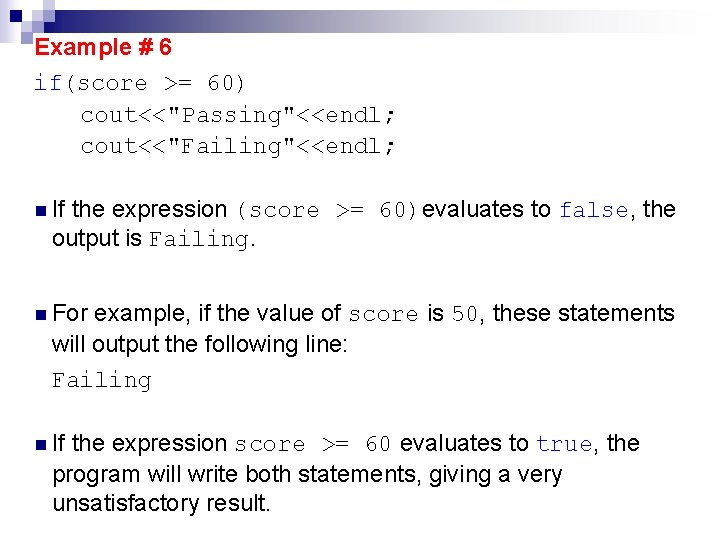Example # 6 if(score >= 60) cout<<"Passing"<<endl; cout<<"Failing"<<endl; n If the expression (score >= 60)evaluates to false, the output is Failing. n For example, if the value of score is 50, these statements will output the following line: Failing n If the expression score >= 60 evaluates to true, the program will write both statements, giving a very unsatisfactory result.if(score >= 60) cout<<"Passing"<<endl; cout<<"Failing"<<endl; n If the value of score is 70, these statements will output the following lines: Passing Failing The correct code to print Passing or Failing, depending on the value of score, is if(score >= 60) cout<<"Passing"<<endl; else cout<<"Failing"<<endl;Example # 7 if(age > 18) { cout<<" Eligible to vote. "<<endl; cout<<" No longer a minor. "<<endl; } else { cout<<"Not eligible to vote. "<<endl; cout<<"Still a minor. "<<endl; } What would happen if there is no braces ? !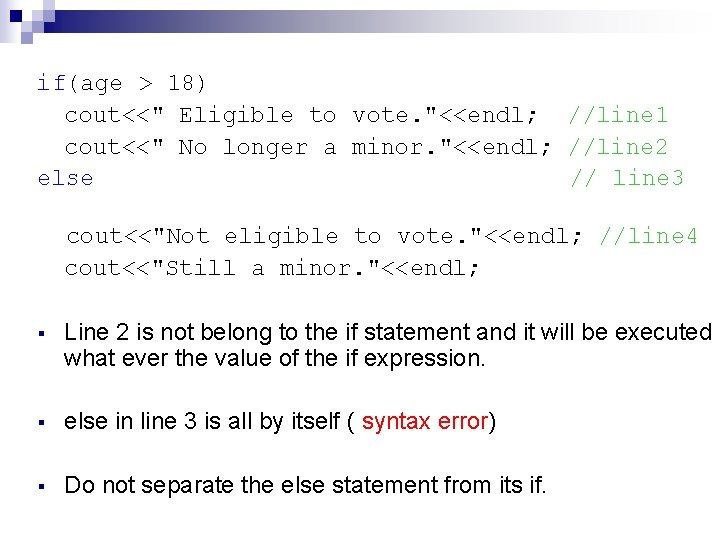if(age > 18) cout<<" Eligible to vote. "<<endl; //line 1 cout<<" No longer a minor. "<<endl; //line 2 else // line 3 cout<<"Not eligible to vote. "<<endl; //line 4 cout<<"Still a minor. "<<endl; § Line 2 is not belong to the if statement and it will be executed what ever the value of the if expression. § else in line 3 is all by itself ( syntax error) § Do not separate the else statement from its if.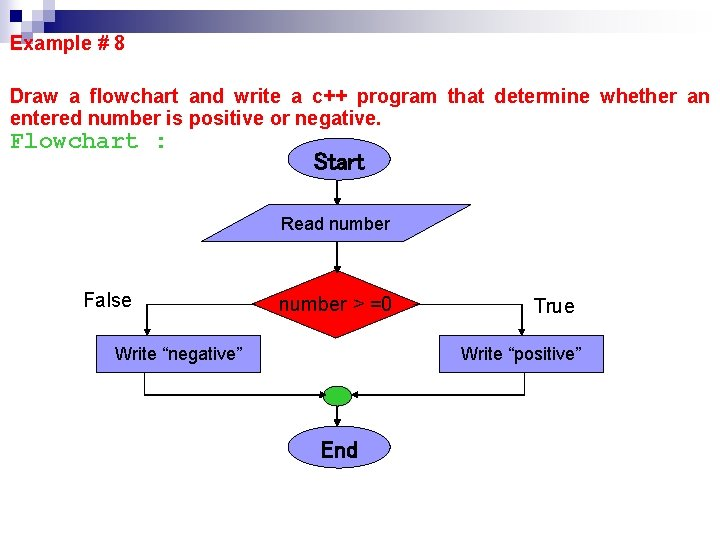Example # 8 Draw a flowchart and write a c++ program that determine whether an entered number is positive or negative. Flowchart : Start Read number False number > =0 Write “negative” True Write “positive” End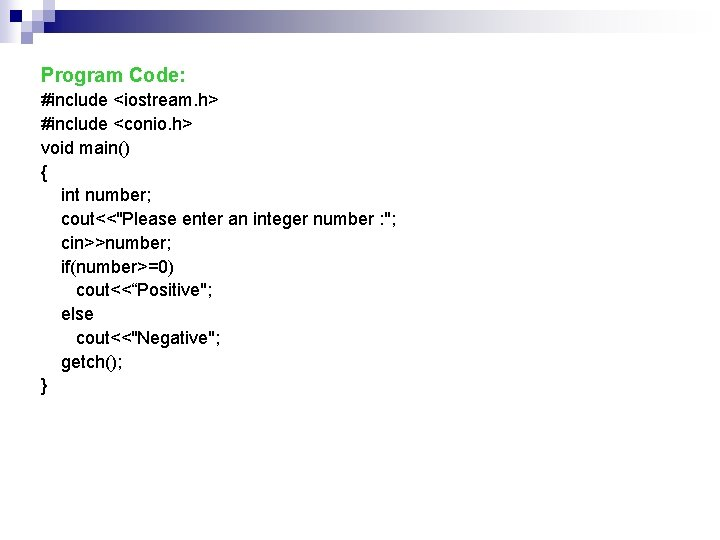Program Code: #include <iostream. h> #include <conio. h> void main() { int number; cout<<"Please enter an integer number : "; cin>>number; if(number>=0) cout<<“Positive"; else cout<<"Negative"; getch(); }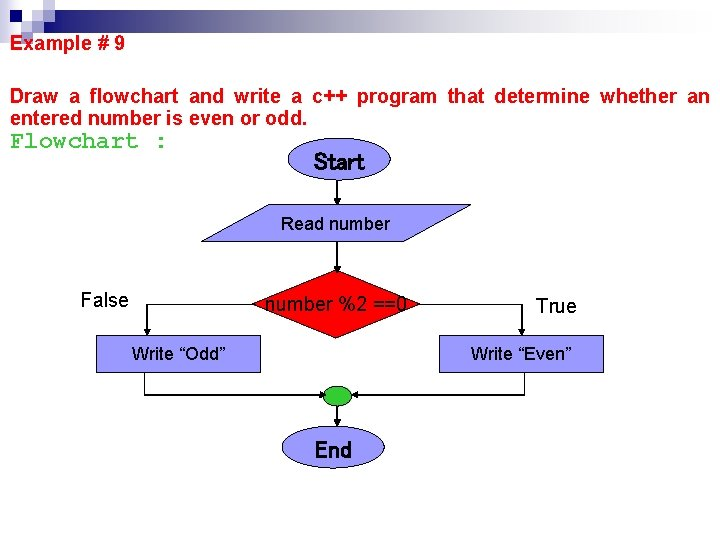Example # 9 Draw a flowchart and write a c++ program that determine whether an entered number is even or odd. Flowchart : Start Read number False number %2 ==0 Write “Odd” True Write “Even” End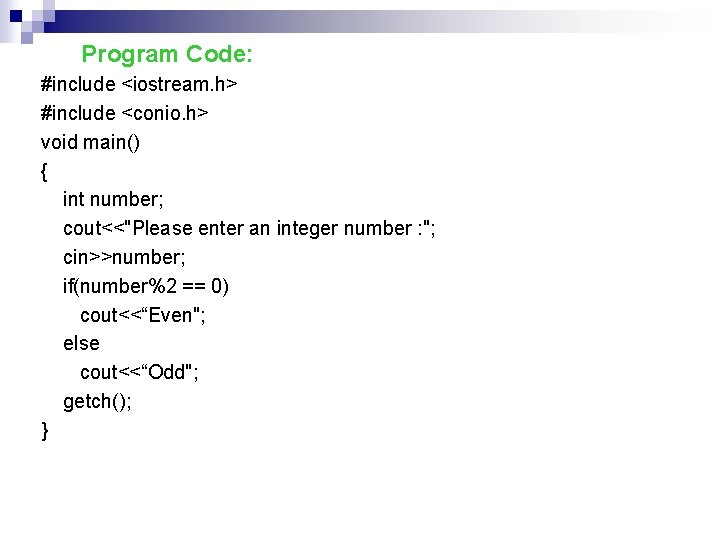Program Code: #include <iostream. h> #include <conio. h> void main() { int number; cout<<"Please enter an integer number : "; cin>>number; if(number%2 == 0) cout<<“Even"; else cout<<“Odd"; getch(); }Example # 10 Draw a flowchart and write a c++ program that reads an integer number of two digits, then display each digit in a separate line after checking that the number consists of only two digits, otherwise display an appropriate message. Flowchart : Start Read number False 10<=number <100 True d 1=number/10 d 2=number%10 Write “Wrong Entry” Write d 1, d 2 EndProgram Code: #include <iostream. h> #include <conio. h> void main() { int number; int d 1, d 2; cout<<"Please enter an integer number of two digits : "; cin>>number; if((number>=10) && (number<100)) { d 1=number/10; d 2=number%10; cout<<d 1<<endl<<d 2; } else cout<<"Wrong Entry"; getch(); }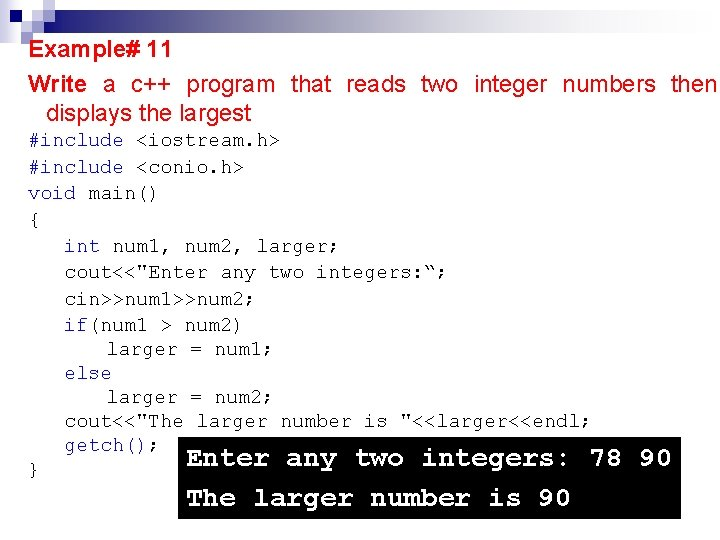Example# 11 Write a c++ program that reads two integer numbers then displays the largest #include <iostream. h> #include <conio. h> void main() { int num 1, num 2, larger; cout<<"Enter any two integers: “; cin>>num 1>>num 2; if(num 1 > num 2) larger = num 1; else larger = num 2; cout<<"The larger number is "<<larger<<endl; getch(); Enter any two integers: 78 } The larger number is 90 90Example # 12 Draw a flowchart and write a c++ program that reads an employee weekly working hours and pay rate, then determine the weekly salary for that employee using time and half for working hours over 40. Flowchart : Start Read hours, rate False hours > 40 salary = 40*rate True salary = 40*rate+(hours-40)*1. 5*rate Write salary EndProgram code: #include <iostream. h> #include <conio. h> void main () { float salary, rate, hours; cout<<"Enter working hours and rate: "; cin>>hours>>rate; if(hours > 40. 0) salary = 40. 0*rate + (hours-40)*1. 5*rate; else salary = hours * rate; cout<<"The salary are "<< salary; getch(); } Enter working hours and rate: 46 10 The salary are 490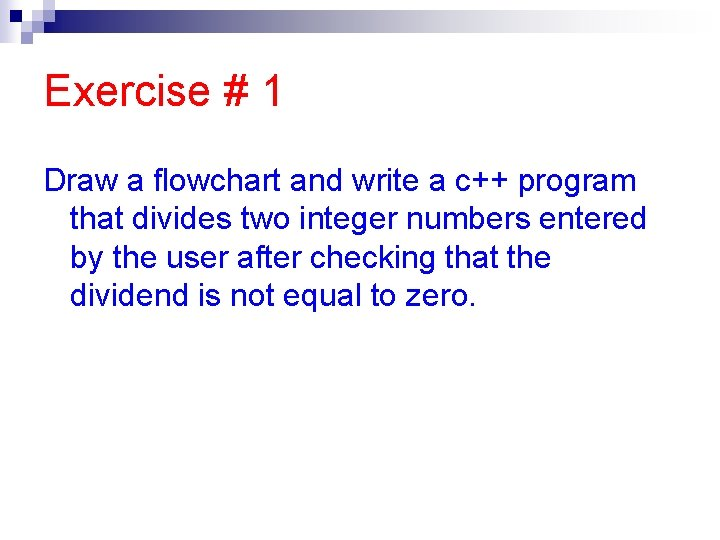Exercise # 1 Draw a flowchart and write a c++ program that divides two integer numbers entered by the user after checking that the dividend is not equal to zero.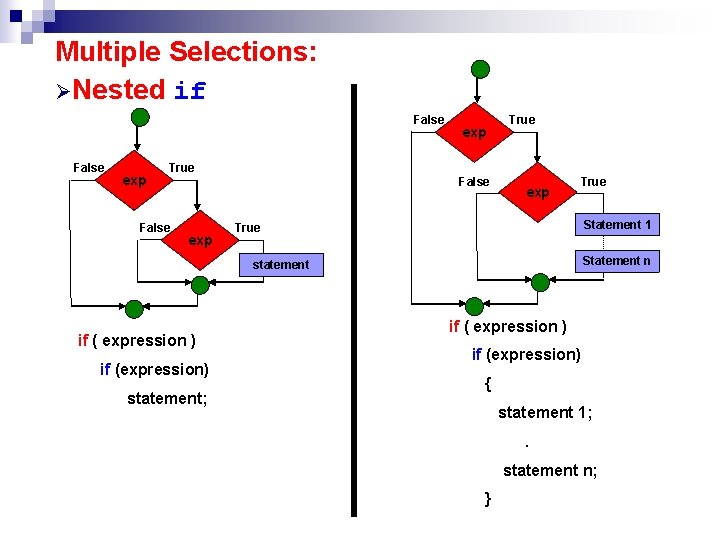Multiple Selections: ØNested if False exp True Statement 1 True Statement n statement if ( expression ) if (expression) statement; if ( expression ) if (expression) { statement 1; . statement n; }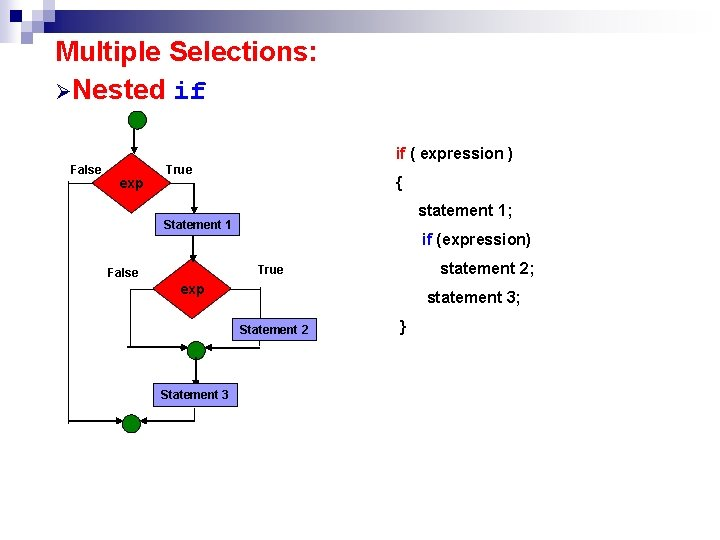Multiple Selections: ØNested if if ( expression ) False exp True { statement 1; Statement 1 if (expression) statement 2; True False exp statement 3; Statement 2 Statement 3 }Multiple Selections: ØNested if/else if ( expression ) if (expression) False statement 1; True exp else False statement 4 exp True statement 3 False statement 2 exp True statement 1 statment 2; else if (expression) statement 3; else statment 4; q Not every if must have an else q It could be multiple nested ifMultiple Selections: ØNested if/else False exp statement 1 True False exp statement 3 if ( expression ) if (expression) statement 2; else statement 3; else statement 1; False True statement 2 False exp True exp statement 3 statement 2 if ( expression ) statment 1; else if (expression) statement 2; else statment 3; statement 1Multiple Selections: ØNested if and if/else False exp True False exp statement 2 if ( expression ) if (expression) False True exp statement 2 False exp statement 1 True statement 1 if ( expression ) { statement 1; if (expression) else statement 2; True statement 1; } else statement 2;Example# 13 Write a c++ program that reads a student mark then determine his grade (A, B, C, D, or F).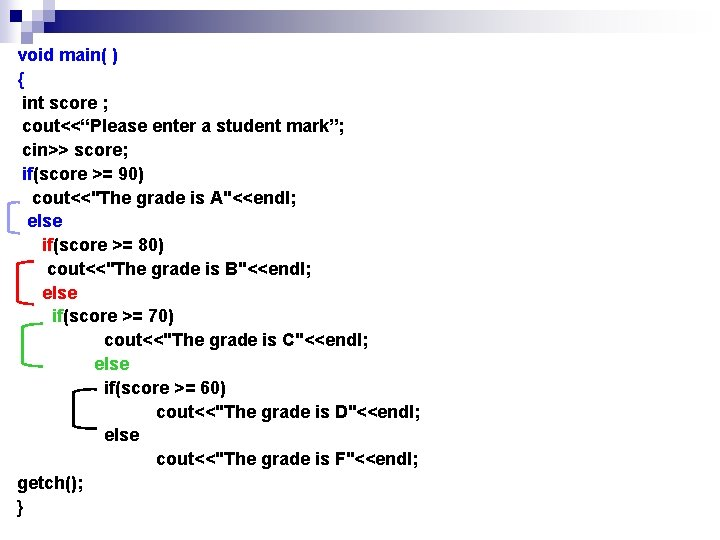void main( ) { int score ; cout<<“Please enter a student mark”; cin>> score; if(score >= 90) cout<<"The grade is A"<<endl; else if(score >= 80) cout<<"The grade is B"<<endl; else if(score >= 70) cout<<"The grade is C"<<endl; else if(score >= 60) cout<<"The grade is D"<<endl; else cout<<"The grade is F"<<endl; getch(); }the previous code can be rewritten as follows: void main() { int score ; cout<<“Please enter a student mark”; cin>> score; if(score >= 90) cout<<"The grade is A"<<endl; else if(score >= 80) cout<<"The grade is B"<<endl; else if(score >= 70) cout<<"The grade is C"<<endl; else if(score >= 60) cout<<"The grade is D"<<endl; else cout<<"The grade is F"<<endl; getch(); }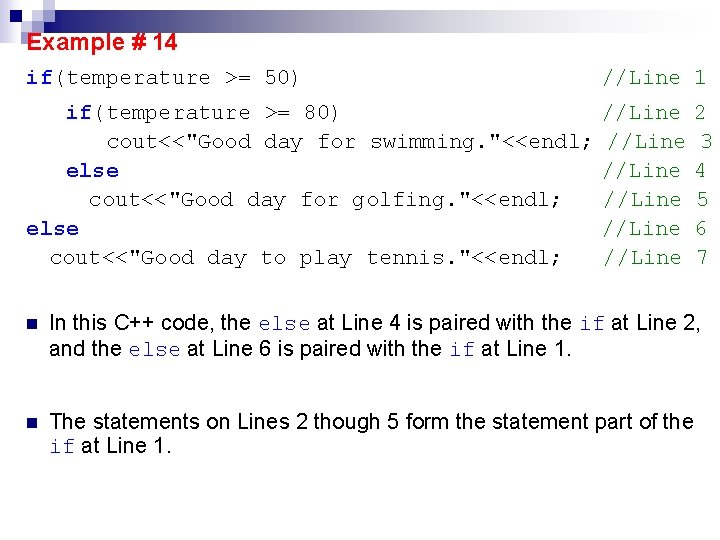Example # 14 if(temperature >= 50) //Line 1 if(temperature >= 80) cout<<"Good day for swimming. "<<endl; else cout<<"Good day for golfing. "<<endl; else cout<<"Good day to play tennis. "<<endl; //Line //Line 2 3 4 5 6 7 n In this C++ code, the else at Line 4 is paired with the if at Line 2, and the else at Line 6 is paired with the if at Line 1. n The statements on Lines 2 though 5 form the statement part of the if at Line 1.Example #15 if(temperature >= if(temperature cout<<"Good else cout<<"Good 70) //Line 1 >= 80) //Line 2 day for swimming. "<<endl; //Line 3 //Line 4 day for golfing. "<<endl; //Line 5 n For the else at Line 4, it is paired with the most recent incomplete if at Line 2. n In this code, the if at Line 1 has no else and is a one-way selection.Example # 16 if(GPA >= 2. 0) //Line if(GPA >= 3. 9) //Line cout<<" graduated with honor. "<<endl; //Line else //Line cout<<"Current GPA below graduation requirement. <<"n. See your academic advisor. "<<endl; //Line 1 2 3 4 " 5 n Following the rule of pairing an else with an if, the else at Line 4 is paired with the if at Line 2. n This pairing produces a very unsatisfactory result. n Suppose that GPA is 3. 8. The expression in the if at Line 1 evaluates to true, and the statement part of the if, which is an if. . . else structure, executes.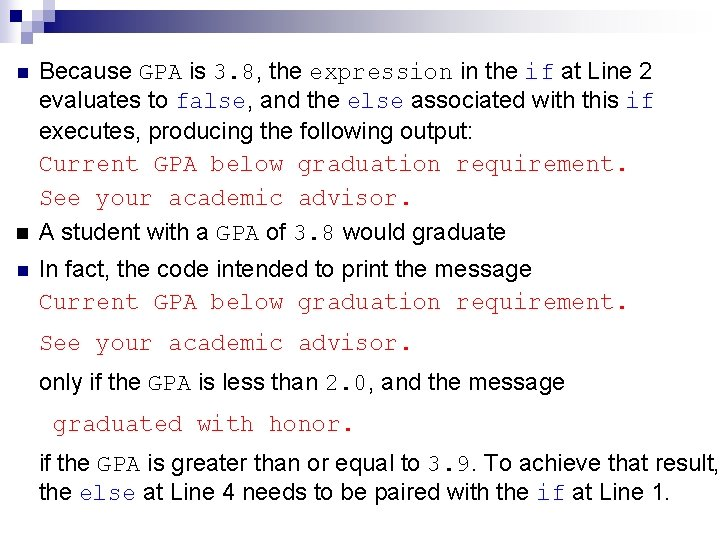n Because GPA is 3. 8, the expression in the if at Line 2 evaluates to false, and the else associated with this if executes, producing the following output: Current GPA below graduation requirement. See your academic advisor. A student with a GPA of 3. 8 would graduate n In fact, the code intended to print the message Current GPA below graduation requirement. See your academic advisor. only if the GPA is less than 2. 0, and the message graduated with honor. if the GPA is greater than or equal to 3. 9. To achieve that result, the else at Line 4 needs to be paired with the if at Line 1.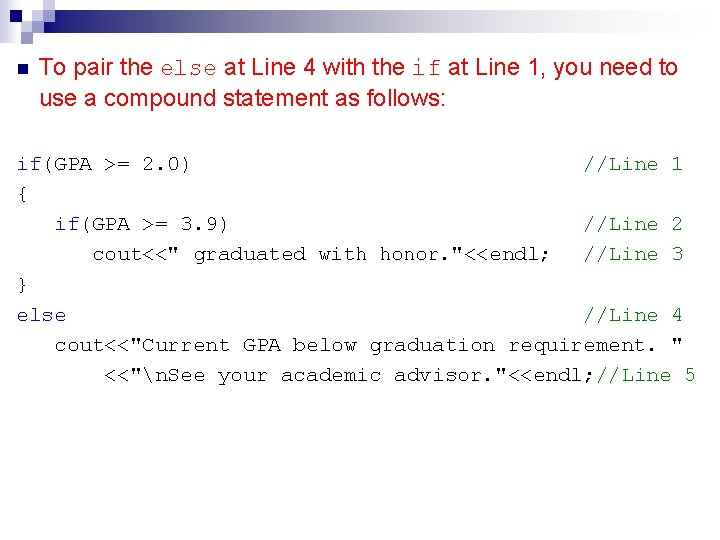n To pair the else at Line 4 with the if at Line 1, you need to use a compound statement as follows: if(GPA >= 2. 0) //Line 1 { if(GPA >= 3. 9) //Line 2 cout<<" graduated with honor. "<<endl; //Line 3 } else //Line 4 cout<<"Current GPA below graduation requirement. " <<"n. See your academic advisor. "<<endl; //Line 5Example # 17 What is the output of the following code segment: int age; cin>>age; if (age>=5) if (age<=11) cout<<“child”; else cout<<“teenager”; ØInput: 6 Output: child ØInput: 15 Output: teenager ØInput: 4 Output: Nothing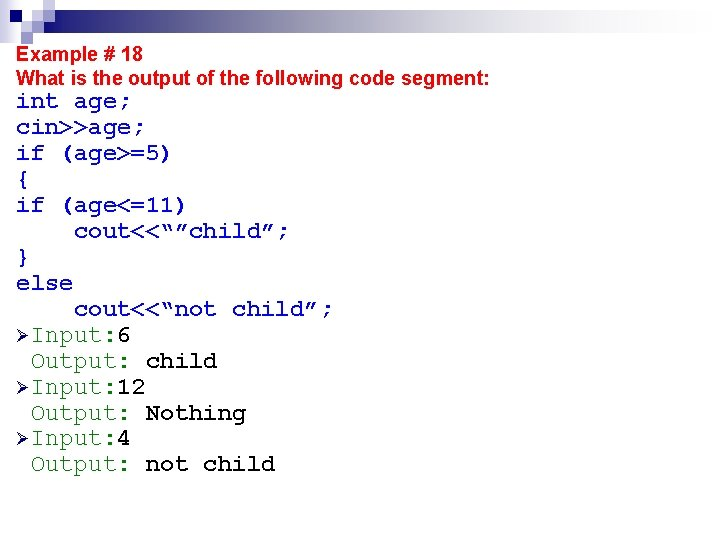Example # 18 What is the output of the following code segment: int age; cin>>age; if (age>=5) { if (age<=11) cout<<“”child”; } else cout<<“not child”; ØInput: 6 Output: child ØInput: 12 Output: Nothing ØInput: 4 Output: not child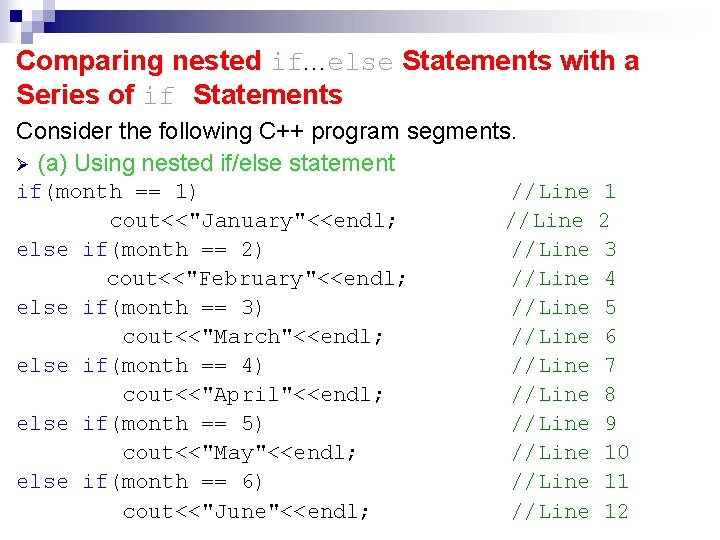Comparing nested if. . . else Statements with a Series of if Statements Consider the following C++ program segments. Ø (a) Using nested if/else statement if(month == 1) cout<<"January"<<endl; else if(month == 2) cout<<"February"<<endl; else if(month == 3) cout<<"March"<<endl; else if(month == 4) cout<<"April"<<endl; else if(month == 5) cout<<"May"<<endl; else if(month == 6) cout<<"June"<<endl; //Line //Line //Line 1 2 3 4 5 6 7 8 9 10 11 12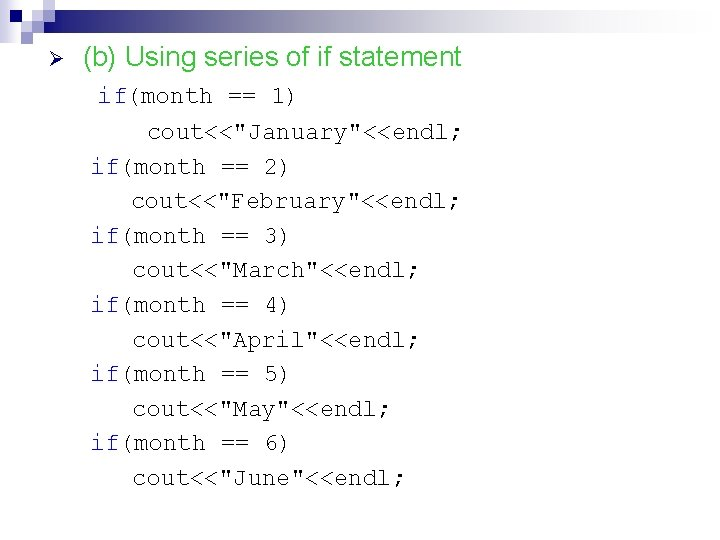Ø (b) Using series of if statement if(month == 1) cout<<"January"<<endl; if(month == 2) cout<<"February"<<endl; if(month == 3) cout<<"March"<<endl; if(month == 4) cout<<"April"<<endl; if(month == 5) cout<<"May"<<endl; if(month == 6) cout<<"June"<<endl;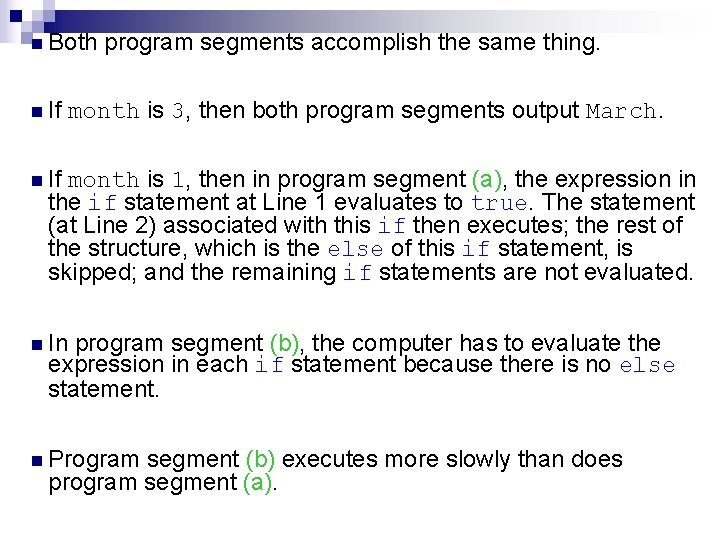n Both n If program segments accomplish the same thing. month is 3, then both program segments output March. n If month is 1, then in program segment (a), the expression in the if statement at Line 1 evaluates to true. The statement (at Line 2) associated with this if then executes; the rest of the structure, which is the else of this if statement, is skipped; and the remaining if statements are not evaluated. n In program segment (b), the computer has to evaluate the expression in each if statement because there is no else statement. n Program segment (b) executes more slowly than does program segment (a).Example # 19 Write a c++ program that reads two integer numbers then finds the largest, or display an appropriate message if they are equal. void main() { int num 1, num 2; cout<<"Enter any two integers: ”; cin>>num 1>>num 2; cout<<endl; if(num 1 > num 2) cout<<"The larger number is"<<num 1<<endl; else if(num 2 > num 1) cout<<"The larger number is "<<num 2<<endl; else cout<<"Both numbers are equal. "<<endl; getch(); }Example # 20 Write a c++ program that reads a character from the user then finds whether the entered character is an alpabetic, numberic, or a symbol. #include <iostream. h> #include <conio. h> #include <ctype. h> void main ( ) { char input; cout <<"Enter any character: "; cin>>input; int code=toascii(input); cout<<input<<"has ASCII code="<<code<<'n'; if( isalpha ( input ) ) cout << "Alphabetic charactern"; else if ( isdigit ( input ) ) cout << "Numeric digitn"; else cout<<“A symboln”; getch(); }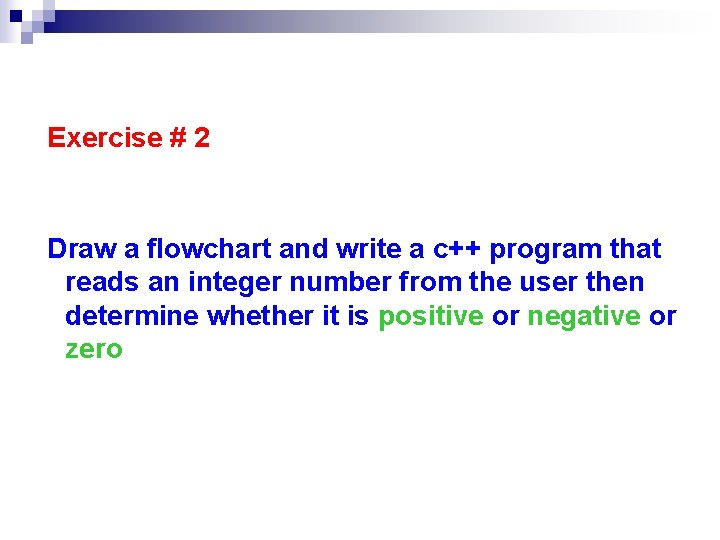Exercise # 2 Draw a flowchart and write a c++ program that reads an integer number from the user then determine whether it is positive or negative or zero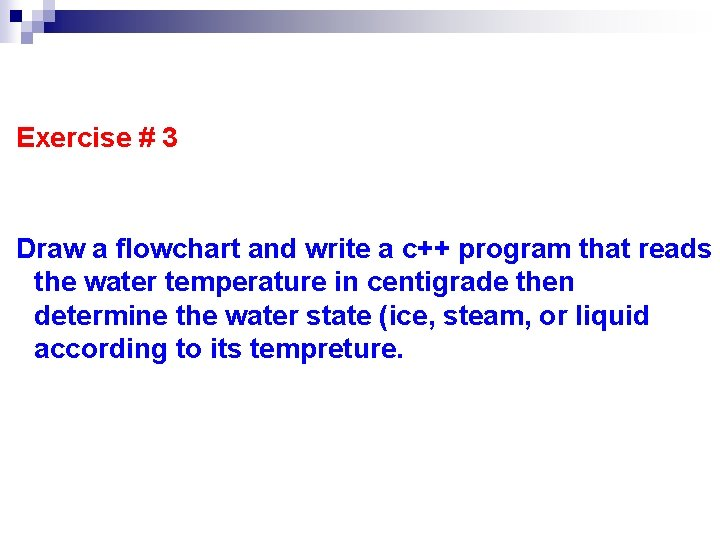Exercise # 3 Draw a flowchart and write a c++ program that reads the water temperature in centigrade then determine the water state (ice, steam, or liquid according to its tempreture.